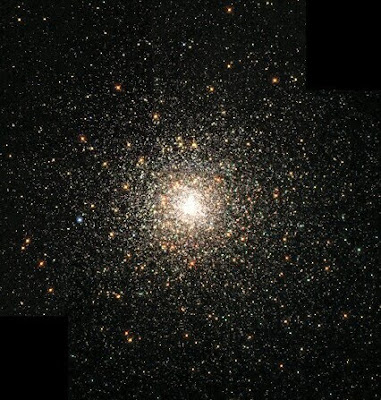## Monday, November 1, 2010

### Basic Problems in Astrophysics (2)An interesting problem is to obtain the distance modulus for a typical star in a globular cluster.

One of the more basic and important quantities in astrophysics is the "distance modulus" - so one would like to see how it is obtained and how used. This is actually a useful measure of the actual rate of emission of visible radiation by a star, and depends on the star’s actual distance. It is often denoted as (m – M) or the difference between the apparent magnitude of a star, and its absolute magnitude M.

The "apparent magnitude" is the apparent brightness registered on a logarithmic scale. For example, the Sun's apparent magnitude is a whopping (-26.5) [Note: the more negative the value, the greater the brightness, the more positive the lower]. Now, the brightest star we can see is Sirius which has an apparent magnitude of about (-1.6). The ratio of brightness is computed on the basis that every five magnitudes registers 100 times brightness ratio. Thus, if star A is at a magnitude of +3 and star B at +8, then star A is brighter than star B by:

(2.512)^5 ~ 100

or 100 times.

(Since on the stellar magnitude scale each single magnitude difference rates as a brightness ratio of 2.512 times).

Thus, on the apparent magnitude scale, the Sun is brighter than Sirius by about 25 magnitudes, for a brightness ratio of:

(2.512)^25 = 10^10

or about ten billion times! So merely casually glancing at the stars (or Sun) gives no insight into how bright they really are. (If it did, everyone would assert the Sun is the ‘king’ of stellar creation – but they’d be flat wrong )

But this is an illusion, which is why astronomers choose to use the absolute magnitude (M). In terms of absolute magnitudes, the standard distance to compare all stellar outputs is exactly 10 parsecs, or 32.6 light years. Thus, to ascertain the Sun’s absolute magnitude it must be “moved” to ten parsecs and its brightness re-assessed.

Of course, one needn’t actually move the Sun to do this – merely use the inverse square light intensity law, e.g. the intensity or brightness of a light source is proportional to the inverse square of the distance.

Thus, the Sun, at nearly magnitude (-26.5) where it sits at 1.5 x 10^8 km, but at 32.6 light years, it will be at a distance of 3.08 x 10^14 km or about 2.06 million times further away. Hence, one must reduce its apparent brilliance by the inverse of that factor squared,or:

(1/ 2.06 x 10^6)^2 = 2.3 x 10^-13

Thus, its brightness diminishes by more than 30 orders or magnitude (recall that the stellar magnitude scale is a logarithmic one).

Now, let L(d) be the observed light from a star at its actual distance d, and let L(10) be the amount of light we’d receive if it were at 10 parsecs.

From the definition of stellar magnitudes, we have:

m – M = 2.5 log (L(10)/ L(d))

where the ‘2.5’ factor reminds us that for every magnitude difference, the ratio of light difference is ~2.5: 1.From the inverse square law for light:

L(10)/ L(d) = (d/ 10)^2

Now, combine the two equations by substituting the last into the first (e.g for L(10)/L(d):

m - M = 5 log (d/ 10)

so that 5 log (d/10) is the distance modulus and it shows we only need to know the difference in magnitudes, m – M to find the distance d.

By example, what if (m – M) = 10 magnitudes?

Then: 10 = 5 log (d/10)2 = log (d/ 10)

or, 10^2 = 100

= d/ 10-> d = 1000 pc